# Midsegment of a Triangle

Midsegment of a Triangle

A closed figure made with 3 line segments forms the shape of a triangle.

Let us explore the world of triangle

Triangle has many subparts. Such as, angles, sides, median, midpoint, midsegment, etc. Here's an activity for you. You can now visualize various types of triangles in math based on their sides and angles. Try changing the position of the vertices to understand the relationship between sides and angles of a triangle.

In the later part of this chapter we will discuss about midpoint and midsegments of a triangle.

Given any two points, say $$A$$ and $$C$$, the midpoint is a point $$B$$ which is located halfway between the points $$A$$ and $$B$$.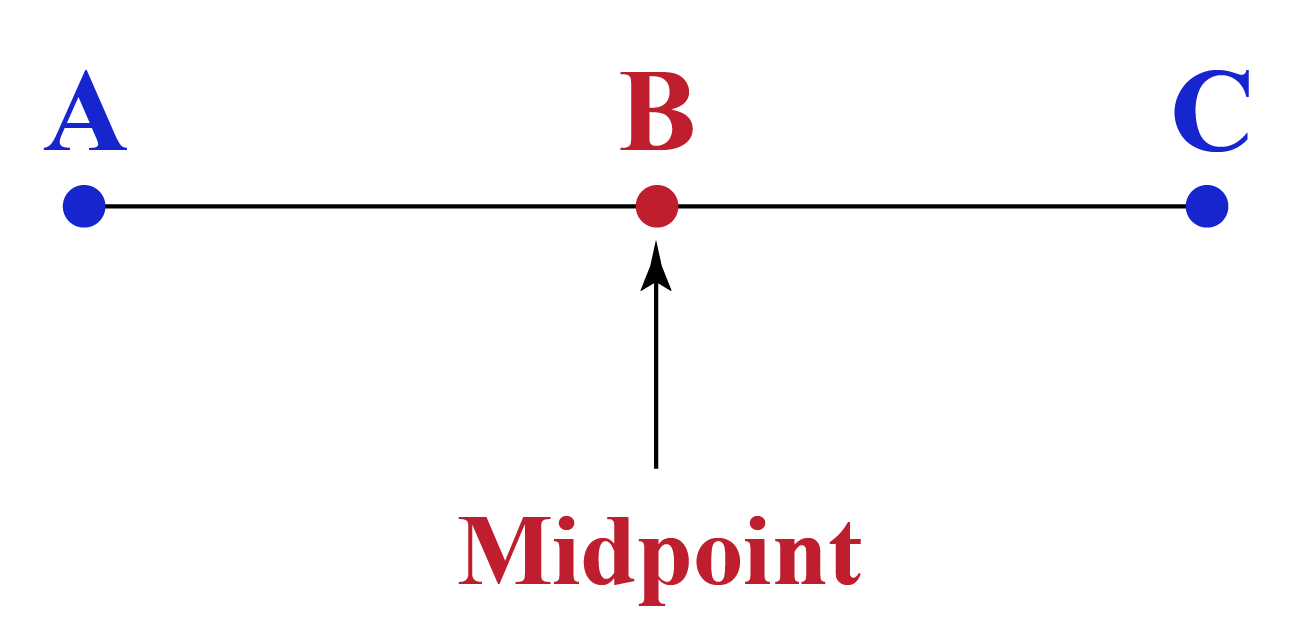Observe that the point $$B$$ is equidistant from $$A$$ and $$C$$.

A midpoint exists only for a line segment.

Line which connects the midpoint is termed as midsegment.

In this mini-lesson, we will explore the world of midsegment of a triangle by finding the answers to the questions like what is midsegment of a triangle, triangle midsegment theorem, and proof with the help of interactive questions.

So let's get started!

## Lesson Plan

 1 What Is Midsegment of a Triangle? 2 Important Notes on Midsegment of a Triangle 3 Solved Examples on Midsegment of a Triangle 4 Interactive Questions on Midsegment of a Triangle 5 Challenging Question on Midsegment of a Triangle

## What Is Midsegment of a Triangle?

### Midsegment of a Triangle Definition

A midsegment of a triangle is a line segment that joins the midpoints or center of two opposite or adjacent sides of a triangleIn the above figure, D is the midpoint of AB and E is the midpoint of AC.

Here DE is a midsegment of a triangle ABC.

## Triangle Midsegment Theorem

The midsegment theorem states that a line segment connecting the midpoints of any two sides of a triangle is parallel to the third side of a triangle and is half of it.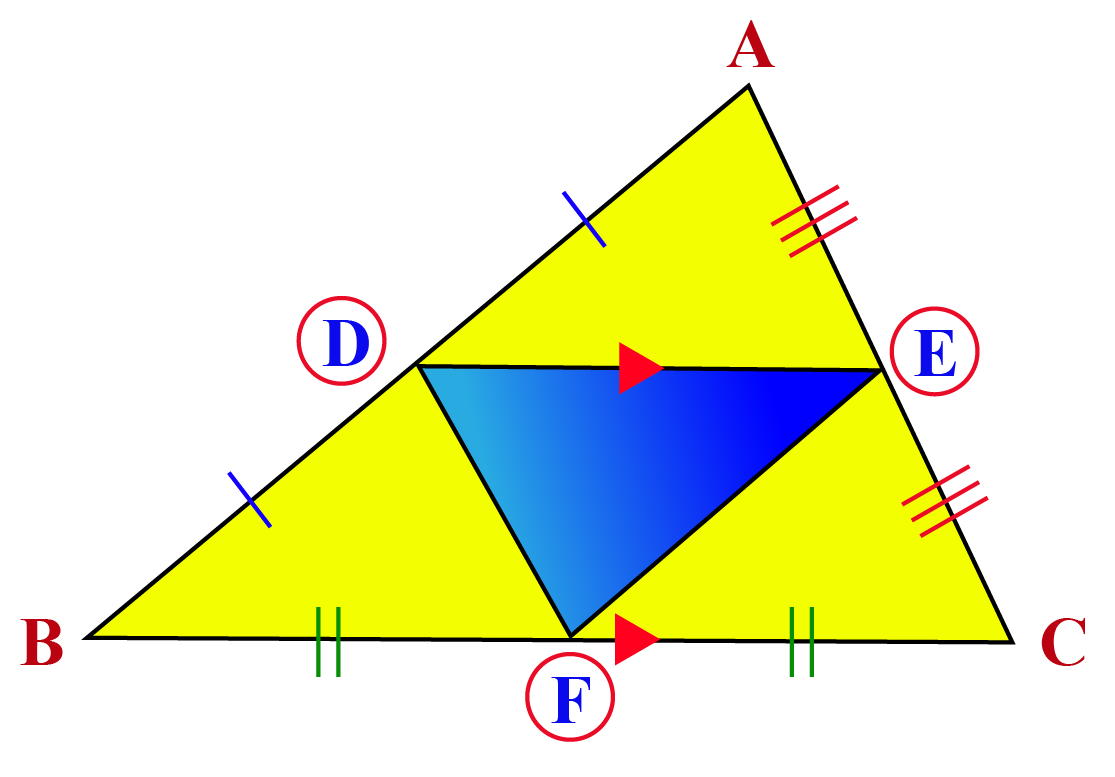In the triangle ABC we have,

$$AD=DB$$  and  $$AE=EC$$

Then according to the midsegment theorem

$$DE∥BC$$ and $$DE=\dfrac{1}{2}\ BC$$

Similarly,

$$AD=DB$$  and  $$BF=FC$$

Then according to the midsegment theorem

$$DF∥AC$$ and $$DF=\dfrac{1}{2}\ AC$$

Similarly,

$$AE=EC$$  and  $$BF=FC$$

Then according to the midsegment theorem

$$EF∥AB$$ and $$EF=\dfrac{1}{2}\ AB$$

Proof for Midsegment of a Triangle

In the above section, we saw a triangle $$ABC$$, with $$D,$$ $$E,$$ and $$F$$ as three midpoints.

We need to prove two things to justify the proof of the triangle midsegment theorem:

• $$DE∥BC$$
• $$DE=\dfrac{1}{2}\ BC$$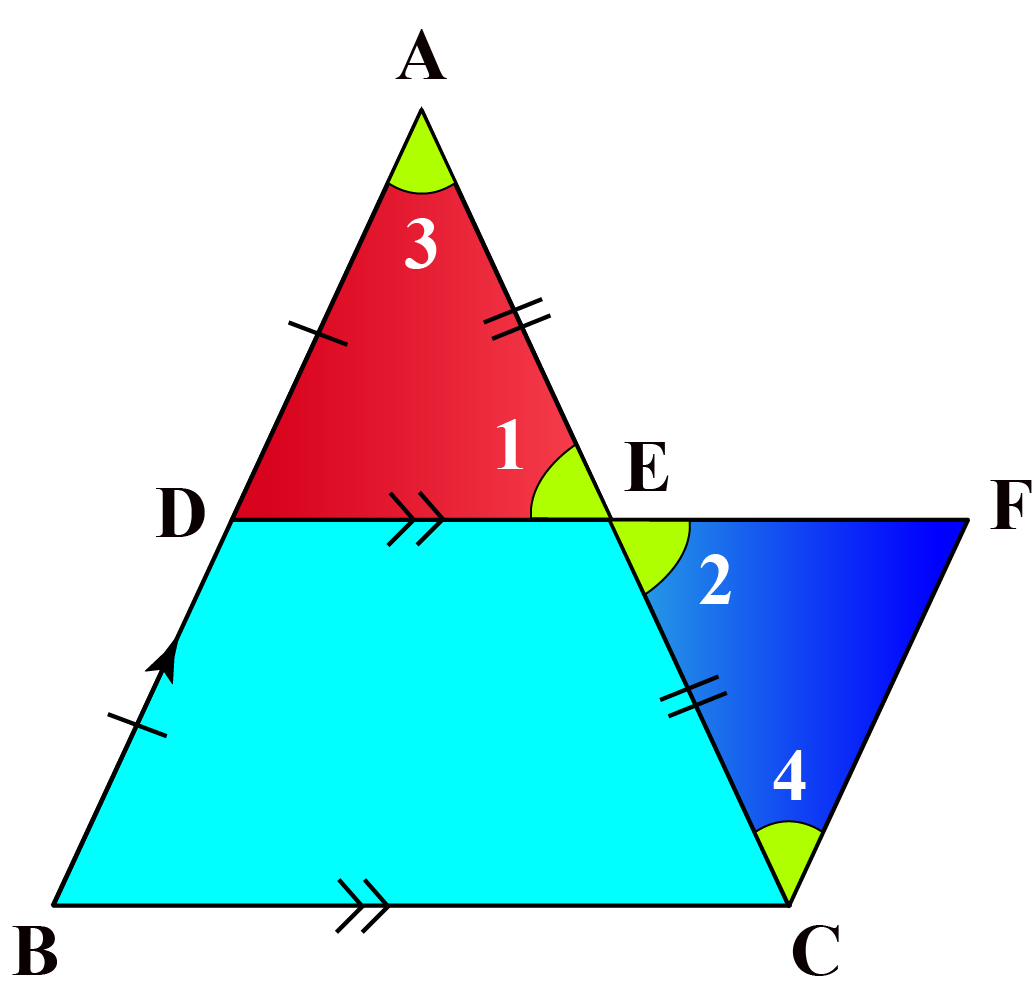Given: D and E are the midpoints of AB and AC

To prove, $$DE∥BC$$ and $$DE=\dfrac{1}{2}\ BC$$ we need to draw a line parallel to AB meet E produced at F.

In $$\bigtriangleup{ADE}$$ and $$\bigtriangleup{CFE}$$

\begin{align} AE &=EC\text{ (E is the midpoint of AC)}\\\ \angle{1} &=\angle{2}\text{ (Vertically opposite angles)}\\\ \angle{3} &=\angle{4}\text{ (Alternate angles)}\end{align}

By AAS congruency of triangle we have,

$$\bigtriangleup{ADE} \cong \bigtriangleup{CFE}$$

By CPCT we have,

$$DE=FE$$

$$AD=CF$$

D is the midpoint of AB

$$AD=BD$$

$$BD=CF$$

DBCF is a parallelogram,

$$DF || BC$$ and $$DF = BC$$

$$DE || BC$$ and $$DF = BC$$

$$DE=\dfrac{1}{2}DF$$

since, DF = BC

$$DE=\dfrac{1}{2}BC$$

Hence Proved

### Midsegment of a Triangle Formula

 Midsegment $$=$$ $$\dfrac{1}{2}\times$$ Triangle Base

## What Is the Converse of the Triangle Midsegment Theorem?

The converse of the midsegment theorem is defined as: When a line segment connects two midpoints of two opposite sides of a triangle and is parallel to the third side of a triangle and is half of it then it is a midsegment of a triangle.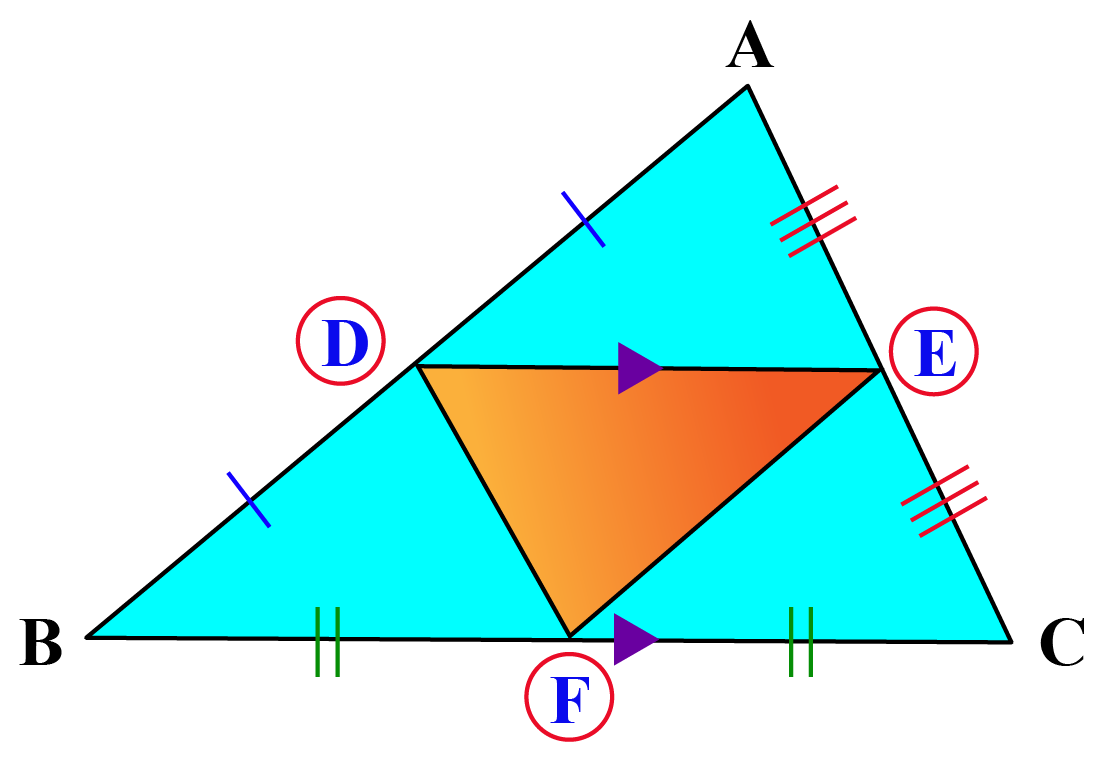In triangle ABC we have,

$$DE∥BC$$ and $$DE=\dfrac{1}{2}\ BC$$

Then according to the converse of the triangle midsegment theorem

$$AD=DB$$ and $$AE=EC$$
$$DE$$ is a midsegment of triangle $$ABC$$

Proof for Converse of the Triangle Midsegment Theorem

In the above section, we saw $$\bigtriangleup{ABC}$$, with $$D,$$ $$E,$$ and $$F$$ as three midpoints.

We need to prove any one of the things mentioned below to justify the proof of the converse of a triangle midsegment theorem:

• $$DE$$ is a midsegment of a $$\bigtriangleup{ABC}$$
• $$AD=DB$$ and $$AE=EC$$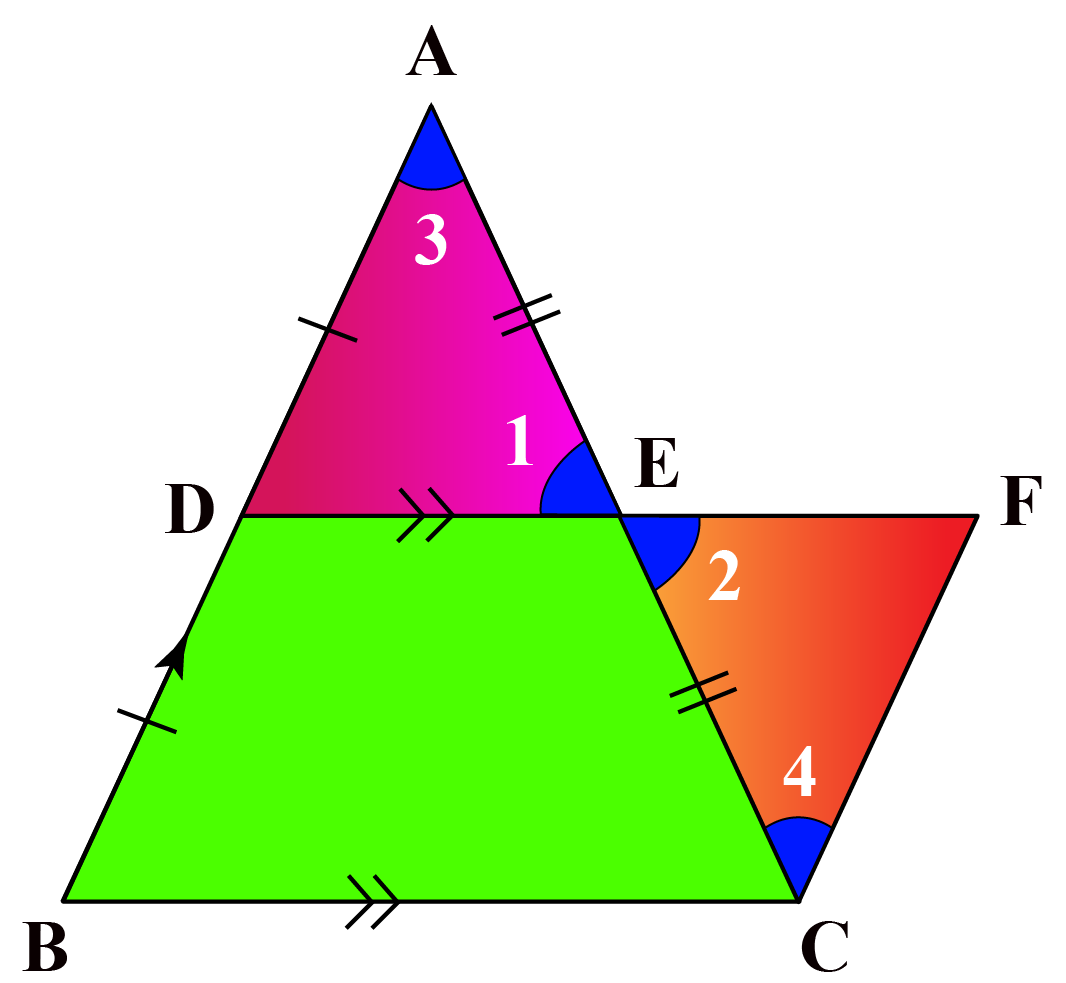We have D as the midpoint of AB, then $$AD = DB$$ and $$DE||BC$$

$$AB$$ $$=$$ $$AD + DB$$ $$=$$ $$DB + DB$$ $$=$$ $$2DB$$

DBCF is a parallelogram.

$$DE||BC$$ and $$BD||CF$$

Opposite sides of a parallelogram are equal.

$$BD=CF$$

$$DA=CF$$

In $$\bigtriangleup{ADE}$$ and $$\bigtriangleup{CFE}$$

\begin{align}\angle{1} &=\angle{2}\text{ (Vertically opposite angles)}\\\ \angle{3} &=\angle{4}\text{ (Alternate angles)}\\\ DA &=CF\end{align}

By AAS congruency of triangle we have,

$$\bigtriangleup{ADE} \cong \bigtriangleup{CFE}$$

By CPCT we have

$$AE=EC$$

E is the midpoint of AC and DF.

Hence, DE is a midsegment of $$\bigtriangleup{ABC}$$.

More Important Topics
Numbers
Algebra
Geometry
Measurement
Money
Data
Trigonometry
Calculus
More Important Topics
Numbers
Algebra
Geometry
Measurement
Money
Data
Trigonometry
Calculus